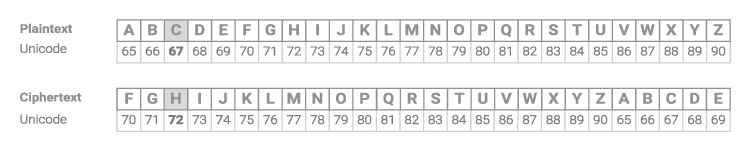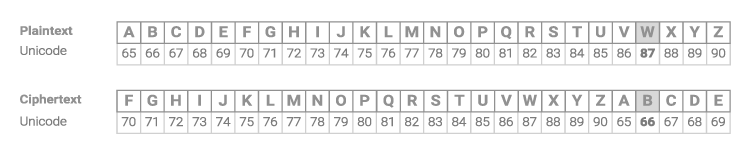Deals Of The Week - hours only!Up to 80% off on all courses and bundles.-Close
Cipher
4. Implementing the cipher – step 1

## Instruction

Amazing! Now we know everything that is necessary to implement the Caesar cipher. We'll start with implementing the cipher for uppercase letters only. In Unicode, the 'A' gets the number 65 and 'Z' gets the number 90. When shifting uppercase letters, we have to stay between these two numbers. Take a look at the examples for shift = 5.letter = 'W'
shift = 5
chr(ord('A') + (ord(letter) - ord('A') + shift) % 26)


A lot of things happened here – let's start from the inside.

The expression ord(letter) - ord('A') finds the offset of a given letter starting with 'A'. The letter 'C' (67) is 2 characters after 'A', and the letter 'W' (87) is 22 characters after 'A'.

Then we add the shift. The letter 'C' (67) with shift 5 should be 7 characters after 'A'. The letter 'W' should be 22 + 5 = 27 characters after 'A'.

But here is a problem: the Unicode character 27 characters after 'A' is not an uppercase letter. It is a character with number 91, uppercase characters run from 65 to 90. This is why we add % 26: this way we will always get a value between 0 and 25, regardless of the shift value.

Next, we simply add the result of modulo to ord('A') (the value of the first uppercase letter) and transform it back.

Finally, we use the chr() function to get the character denoted by the number.

## Exercise

Create a function called cipher() that takes two arguments: an uppercase word and a number that indicates the shift value. The function should return the shifted word (also uppercase).

### Stuck? Here's a hint!

Use the expression below to find the cipher for a given letter:

chr(ord('A') + (ord(letter) - ord('A') + shift) % 26)